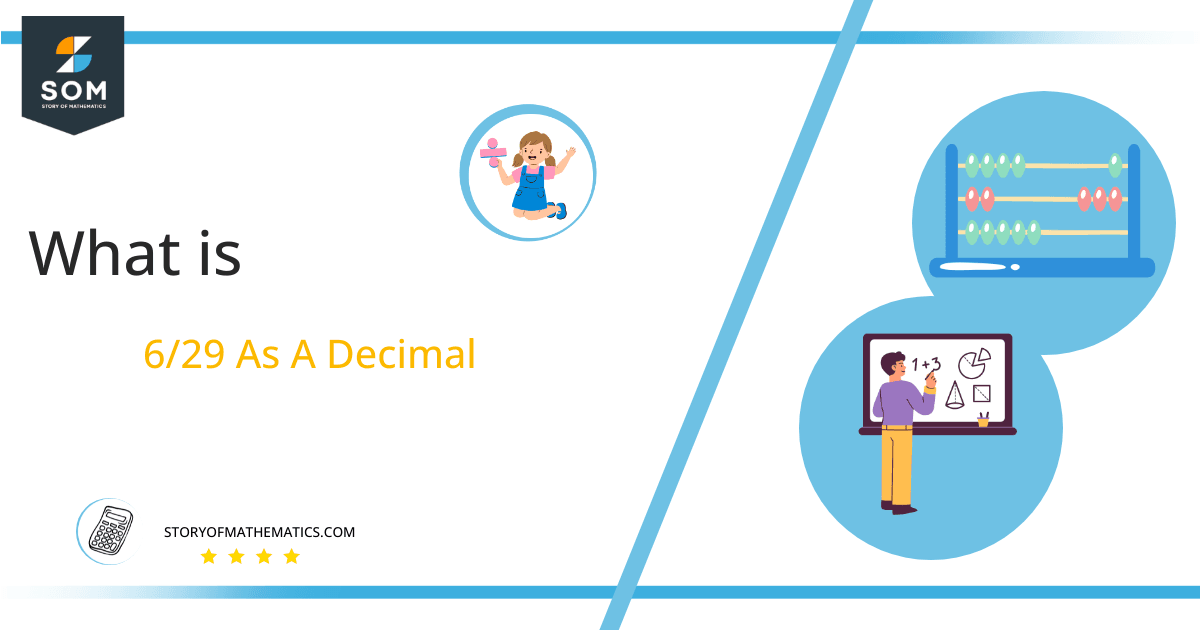# What Is 6/29 as a Decimal + Solution With Free Steps

The fraction 6/29 as a decimal is equal to 0.20689655.

Fractions are a formal representation of the division operation for ease of usage of the user. In Mathematics, fractions are expressed as p/q, where “p” is the numerator or dividend and “q” is the denominator or divisor. The other formal representation of the division’s result is the decimal form that can be achieved by the long division process.Here, we are more interested in the division types that result in a Decimal value, as this can be expressed as a Fraction. We see fractions as a way of showing two numbers having the operation of Division between them that result in a value that lies between two Integers.

Now, we introduce the method used to solve said fraction to decimal conversion, called Long Division, which we will discuss in detail moving forward. So, let’s go through the Solution of fraction 6/29.

## Solution

First, we convert the fraction components, i.e., the numerator and the denominator, and transform them into the division constituents, i.e., the Dividend and the Divisor, respectively.

This can be done as follows:

Dividend = 6

Divisor = 29

We introduce the most important quantity in our division process: the Quotient. The value represents the Solution to our division and can be expressed as having the following relationship with the Division constituents:

Quotient = Dividend $\div$ Divisor = 6 $\div$ 29

This is when we go through the Long Division solution to our problem. The following figure shows the long division:Figure 1

## 6/29 Long Division Method

We start solving a problem using the Long Division Method by first taking apart the division’s components and comparing them. As we have 6 and 29, we can see how 6 is Smaller than 29, and to solve this division, we require that 6 be Bigger than 29.

This is done by multiplying the dividend by 10 and checking whether it is bigger than the divisor or not. If so, we calculate the Multiple of the divisor closest to the dividend and subtract it from the Dividend. This produces the Remainder, which we then use as the dividend later.

Now, we begin solving for our dividend 6, which after getting multiplied by 10 becomes 60.

We take this 60 and divide it by 29; this can be done as follows:

60 $\div$ 29 $\approx$ 2

Where

29 x 2 = 58

This will lead to the generation of a Remainder equal to 60 – 58 = 2. Now this means we have to repeat the process by Converting the 2 into 200  by adding zero in the quotient and multiplying 2 with 10 twice and solving for that:

200 $\div$ 29 $\approx$ 6

Where:

29 x 6 = 174

Therefore, Remainder is equal to 200 – 174 = 26. Now we stop solving this problem, we have a Quotient generated after combining the two pieces of it as 0.206=z, with a Remainder equal to 26.Images/mathematical drawings are created with GeoGebra.